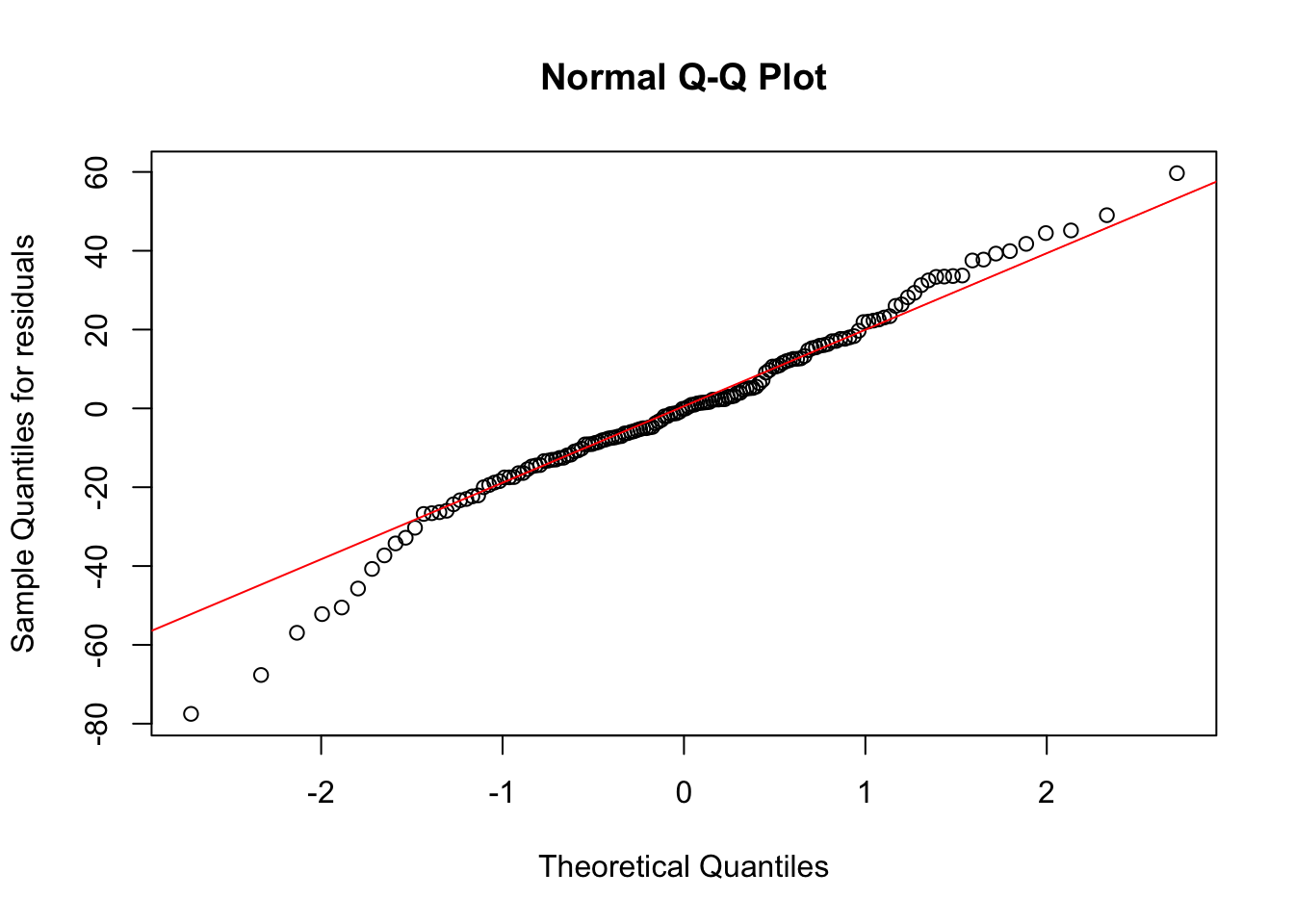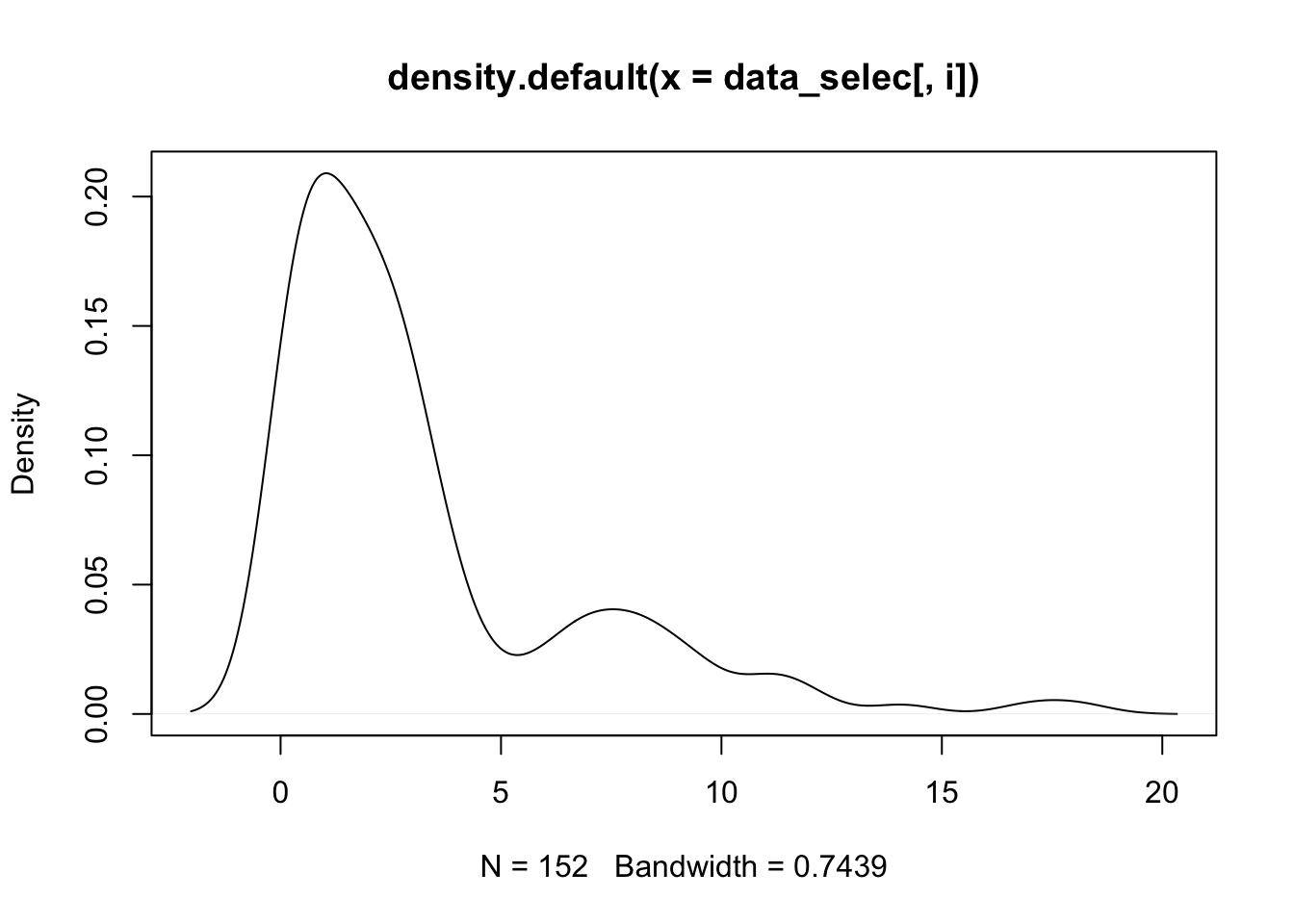The purpose of variable transformation is to make extremely right/left skewed data appear normally distributed. We first check the distribution of the numeric variables we have selected (cadmium, zinc and om).

## PART ONE: variable transformation using log and sqrt

``````library(leaps)
library(sp)
data(meuse)
meuse <- na.omit(meuse)
var_selec <- c("cadmium","zinc","om","ffreq","lime","lead")
## creating the sub
data_selec <- meuse[var_selec]
data_selec\$ffreq <- as.numeric(data_selec\$ffreq)
## replicating previous model
model_1 <- lm(lead~. ,data = data_selec)
summary(model_1)``````
``````##
## Call:
## lm(formula = lead ~ ., data = data_selec)
##
## Residuals:
##     Min      1Q  Median      3Q     Max
## -77.514 -12.536  -0.082  13.660  59.689
##
## Coefficients:
##             Estimate Std. Error t value Pr(>|t|)
## (Intercept)  35.5617     7.0328   5.057 1.26e-06 ***
## cadmium     -13.0918     1.5459  -8.469 2.43e-14 ***
## zinc          0.4300     0.0128  33.606  < 2e-16 ***
## om           -2.3889     0.8193  -2.916 0.004110 **
## ffreq       -10.5112     2.9432  -3.571 0.000481 ***
## lime1       -25.0169     5.8700  -4.262 3.62e-05 ***
## ---
## Signif. codes:  0 '***' 0.001 '**' 0.01 '*' 0.05 '.' 0.1 ' ' 1
##
## Residual standard error: 22.72 on 146 degrees of freedom
## Multiple R-squared:  0.9597, Adjusted R-squared:  0.9584
## F-statistic: 696.1 on 5 and 146 DF,  p-value: < 2.2e-16``````

We check distribution of error and the distribution of the selected variables to determine if we need to do variable transformation

``````qqnorm(residuals(model_1),
ylab="Sample Quantiles for residuals")
qqline(residuals(model_1),
col="red")``````We can see that the residuals are not normally distributed, thus variable transformation is necessary.

``````for (i in 1: 3) {
d <- density(data_selec[,i])
plot(d)
}``````